# Work, Energy & Power

Work done W is defined as the dot product of force F and displacement s.

Work = F.s = Fs cos θ

Here θ is the angle between Force and displacement.

Work done by a variable force: If applied force F is not a constant force, then work done by this force in moving the body from position A to B will be,Here ds is the small displacement.

Units: The unit of work done in S.I is joule (J) and in C.G.S system is erg.

1J = 1 Nm | 1 erg = 1 dyn.cm

1J = 107erg

Power

The rate at which work is done is called power and is defined as,

P = W/t = F.s/v = F.v

Here s is the distance and v is the speed.

Instantaneous power in terms of mechanical energy: 𝑃=𝑑𝐸/𝑑𝑡

Units: The unit of power in S.I system is J/s (watt) and in C.G.S system is erg/s.

Energy

Energy is the ability of the body to do some work. The unit of energy is same as that of work.

Kinetic Energy (KE): It is defined as,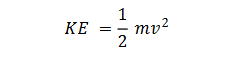Here m is the mass of the body and v is the speed of the body.

Potential Energy (U): Potential energy of a body is defined as, 𝑈=𝑚𝑔

Relation between Kinetic Energy (K) and Momentum (p):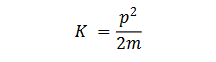Work-Energy Theorem:

It states that work done on the body or by the body is equal to the net change in its Kinetic Energy.

For constant force,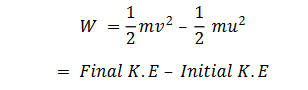Law of conservation of Energy:

It states that, “Energy can neither be created nor destroyed. It can be converted from one form to another. The sum of total energy, in this universe, is always same”.

The sum of the kinetic and potential energies of an object is called mechanical energy. So, E = K+U

In accordance to law of conservation of energy, the total mechanical energy of the system always remains constant.

Coefficient of restitution (e):

It is defined as the ratio between magnitude of impulse during period of restitution to that during period of deformation.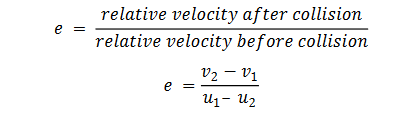Case (i) For perfectly elastic collision, e = 1. Thus, v2 –v1 = u1 –u2. This signifies the relative velocities of two bodies before and after collision are same.

Case (ii) For inelastic collision, e<1. Thus, v2 –v1 < u1 –u2. This signifies, the value of e shall depend upon the extent of loss of kinetic energy during collision.

Case (iii) For perfectly inelastic collision, e = 0. Thus, v2 –v1 =0, or v2 = v1. This signifies the two bodies shall move together with same velocity. Therefore, there shall be no separation between them.

Elastic collision: In an elastic collision, both the momentum and kinetic energy conserved.

Elastic collision in One Dimension:

After collision, the velocity of two body will be,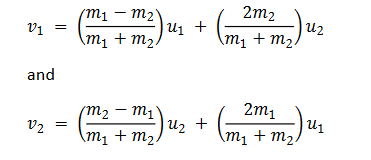Post By : Ravindra Yadav 03 Nov, 2019 1714 views Physics